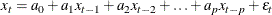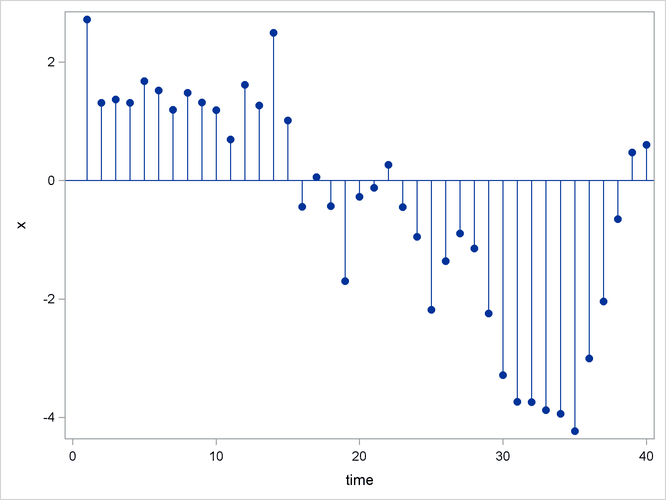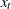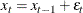# The FORECAST Procedure

### Time Series Methods

Time series models assume the future value of a variable to be a linear function of past values. If the model is a function of past values for a finite number of periods, it is an autoregressive model and is written as follows:The coefficients aare autoregressive parameters. One of the simplest cases of this model is the random walk, where the series dances around in purely random jumps. This is illustrated in Figure 16.10.

Figure 16.10: Random Walk SeriesThevalues are generated by the equationIn this type of model, the best forecast of a future value is the present value. However, with other autoregressive models, the best forecast is a weighted sum of recent values. Pure autoregressive forecasts always damp down to a constant (assuming the process is stationary).

Autoregressive time series models can also be used to predict seasonal fluctuations.